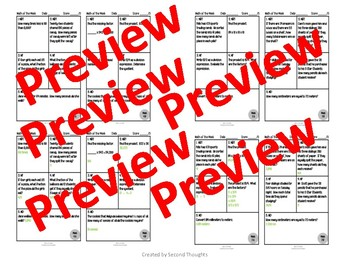# 5th Grade Math of the Week 1st QuarterSubject
Resource Type
File Type

Zip

(2 MB|21 pages)
Standards
• Product Description
• StandardsNEW
This listing is for nine weeks of daily practice or review of 5th grade math standards. This product is great for morning work, small group acceleration or remediation, homework review, test prep, or individual review quick checks. Each week consists of 4 days of problems with 5 problems per day. An answer key is included in the listing for student or teacher use.
Apply and extend previous understandings of multiplication to multiply a fraction or whole number by a fraction.
Interpret a fraction as division of the numerator by the denominator (𝘢/𝘣 = 𝘢 ÷ 𝘣). Solve word problems involving division of whole numbers leading to answers in the form of fractions or mixed numbers, e.g., by using visual fraction models or equations to represent the problem. For example, interpret 3/4 as the result of dividing 3 by 4, noting that 3/4 multiplied by 4 equals 3, and that when 3 wholes are shared equally among 4 people each person has a share of size 3/4. If 9 people want to share a 50-pound sack of rice equally by weight, how many pounds of rice should each person get? Between what two whole numbers does your answer lie?
Write simple expressions that record calculations with numbers, and interpret numerical expressions without evaluating them. For example, express the calculation “add 8 and 7, then multiply by 2” as 2 × (8 + 7). Recognize that 3 × (18932 + 921) is three times as large as 18932 + 921, without having to calculate the indicated sum or product.
Use parentheses, brackets, or braces in numerical expressions, and evaluate expressions with these symbols.
Find whole-number quotients of whole numbers with up to four-digit dividends and two-digit divisors, using strategies based on place value, the properties of operations, and/or the relationship between multiplication and division. Illustrate and explain the calculation by using equations, rectangular arrays, and/or area models.
Total Pages
21 pages
Included
Teaching Duration
N/A
Report this Resource to TpT
Reported resources will be reviewed by our team. Report this resource to let us know if this resource violates TpT’s content guidelines.